# 5TH ANNIVERSARY AUCTION

### Auction Details

Choose Your Layout >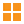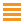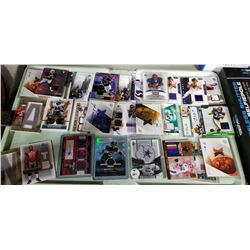101 - Shelf One
CDC Auctions
Shelf One
Bidding Has Concluded

Sold to f***r for (30.00 + 3.90) x 1 = 33.90102 - Shelf One
CDC Auctions
Shelf One
Bidding Has Concluded

Sold to f***r for (80.00 + 10.40) x 1 = 90.40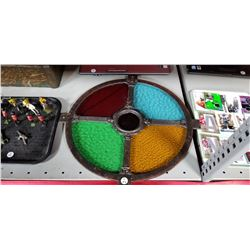103 - Shelf One
CDC Auctions
Shelf One
Bidding Has Concluded

Sold to f***r for (100.00 + 13.00) x 1 = 113.00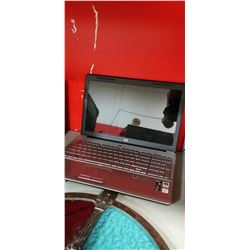104 - Shelf One
CDC Auctions
Shelf One
Bidding Has Concluded

Sold to f***r for (105.00 + 13.65) x 1 = 118.65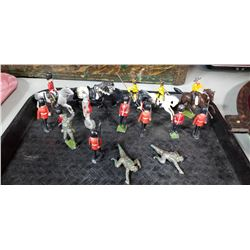105 - Shelf One
CDC Auctions
Shelf One
Bidding Has Concluded

Sold to f***r for (15.00 + 1.95) x 1 = 16.95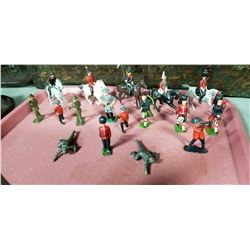106 - Shelf Two
CDC Auctions
Shelf Two
Bidding Has Concluded

Sold to f***r for (25.00 + 3.25) x 1 = 28.25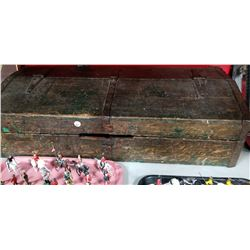107 - Shelf One
CDC Auctions
Shelf One
Bidding Has Concluded

Sold to f***r for (22.50 + 2.93) x 1 = 25.43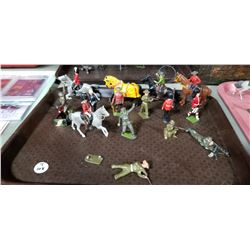108 - Shelf One
CDC Auctions
Shelf One
Bidding Has Concluded

Sold to f***r for (40.00 + 5.20) x 1 = 45.20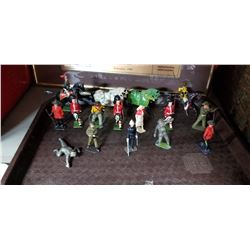109 - Shelf One
CDC Auctions
Shelf One
Bidding Has Concluded

Sold to f***r for (20.00 + 2.60) x 1 = 22.60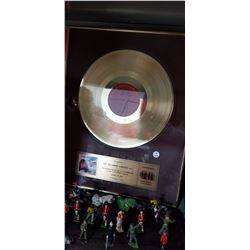110 - Shelf One
CDC Auctions
Shelf One
Bidding Has Concluded

Sold to f***r for (90.00 + 11.70) x 1 = 101.70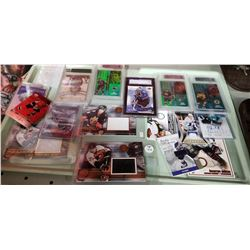111 - Shelf One
CDC Auctions
Shelf One
Bidding Has Concluded

Sold to f***r for (50.00 + 6.50) x 1 = 56.50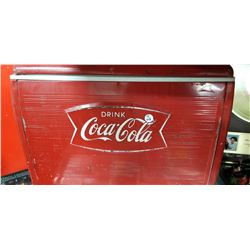112 - Shelf One
CDC Auctions
Shelf One
Bidding Has Concluded

Sold to f***r for (50.00 + 6.50) x 1 = 56.50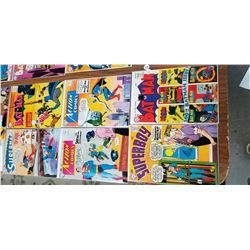113 - Shelf One
CDC Auctions
Shelf One
Bidding Has Concluded

Sold to f***r for (220.00 + 28.60) x 1 = 248.60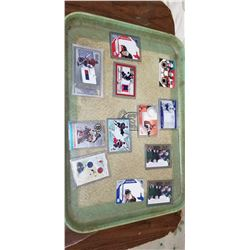114 - Shelf One
CDC Auctions
Shelf One
Bidding Has Concluded

Sold to f***r for (40.00 + 5.20) x 1 = 45.20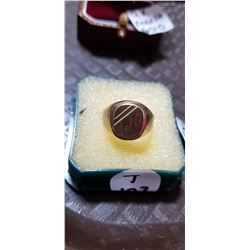115 - Shelf One
CDC Auctions
Shelf One
Bidding Has Concluded

Sold to f***r for (55.00 + 7.15) x 1 = 62.15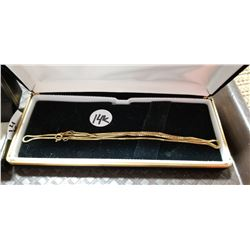116 - Shelf One
CDC Auctions
Shelf One
Bidding Has Concluded

Sold to f***r for (140.00 + 18.20) x 1 = 158.20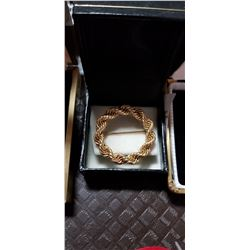117 - Shelf One
CDC Auctions
Shelf One
Bidding Has Concluded

Sold to f***r for (110.00 + 14.30) x 1 = 124.30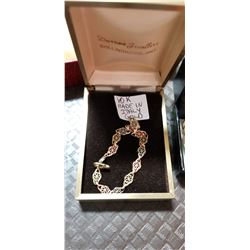118 - Shelf One
CDC Auctions
Shelf One
Bidding Has Concluded

Sold to f***r for (55.00 + 7.15) x 1 = 62.15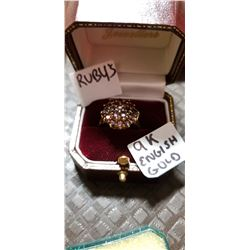119 - Shelf One
CDC Auctions
Shelf One
Bidding Has Concluded

Sold to f***r for (95.00 + 12.35) x 1 = 107.35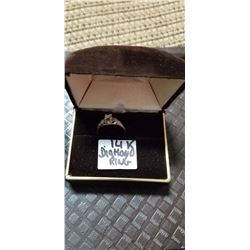121 - Shelf One
CDC Auctions
Shelf One
Bidding Has Concluded

Sold to f***r for (120.00 + 15.60) x 1 = 135.60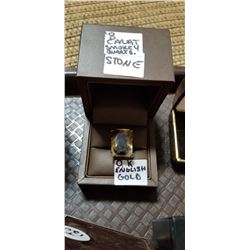122 - Shelf One
CDC Auctions
Shelf One
Bidding Has Concluded

Sold to f***r for (140.00 + 18.20) x 1 = 158.20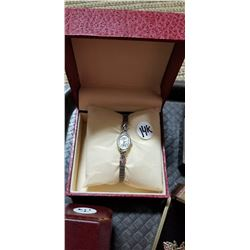123 - Shelf One
CDC Auctions
Shelf One
Bidding Has Concluded

Sold to f***r for (100.00 + 13.00) x 1 = 113.00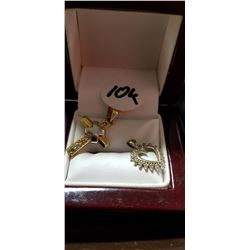125 - Shelf One
CDC Auctions
Shelf One
Bidding Has Concluded

Sold to f***r for (85.00 + 11.05) x 1 = 96.05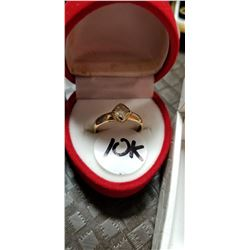126 - Shelf One
CDC Auctions
Shelf One
Bidding Has Concluded

Sold to f***r for (60.00 + 7.80) x 1 = 67.80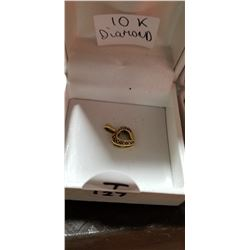127 - Shelf One
CDC Auctions
Shelf One
Bidding Has Concluded

Sold to f***r for (27.50 + 3.58) x 1 = 31.08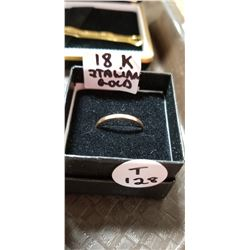128 - Shelf One
CDC Auctions
Shelf One
Bidding Has Concluded

Sold to f***r for (75.00 + 9.75) x 1 = 84.75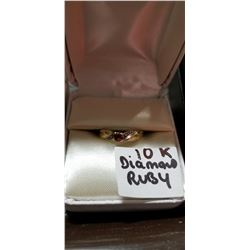129 - Shelf One
CDC Auctions
Shelf One
Bidding Has Concluded

Sold to f***r for (65.00 + 8.45) x 1 = 73.45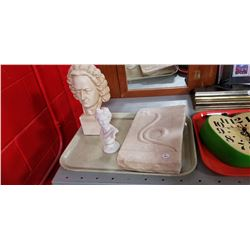131 - Shelf One
CDC Auctions
Shelf One
Bidding Has Concluded

Sold to f***r for (22.50 + 2.93) x 1 = 25.43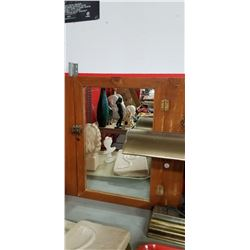132 - Shelf One
CDC Auctions
Shelf One
Bidding Has Concluded

Sold to f***r for (5.00 + 0.65) x 1 = 5.65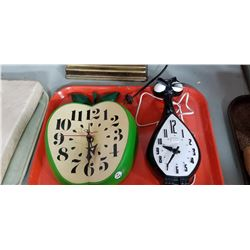133 - Shelf One
CDC Auctions
Shelf One
Bidding Has Concluded

Sold to f***r for (17.50 + 2.28) x 1 = 19.78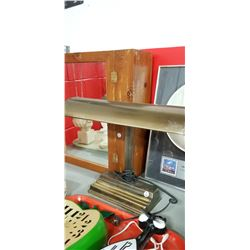134 - Shelf One
CDC Auctions
Shelf One
Bidding Has Concluded

Sold to f***r for (20.00 + 2.60) x 1 = 22.60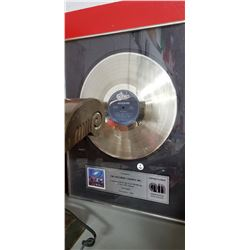135 - Shelf One
CDC Auctions
Shelf One
Bidding Has Concluded

Sold to f***r for (95.00 + 12.35) x 1 = 107.35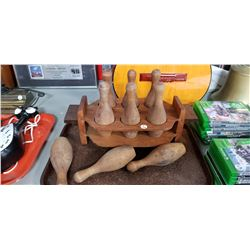136 - Shelf One
CDC Auctions
Shelf One
Bidding Has Concluded

Sold to f***r for (15.00 + 1.95) x 1 = 16.95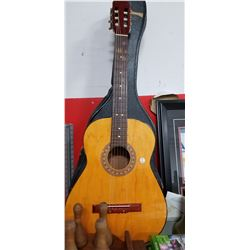137 - Shelf One
CDC Auctions
Shelf One
Bidding Has Concluded

Sold to f***r for (37.50 + 4.88) x 1 = 42.38138 - Shelf One
CDC Auctions
Shelf One
Bidding Has Concluded

Sold to f***r for (35.00 + 4.55) x 1 = 39.55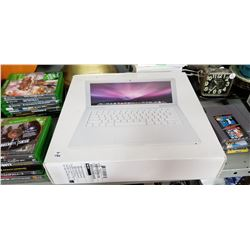140 - Shelf One
CDC Auctions
Shelf One
Bidding Has Concluded

Sold to f***r for (160.00 + 20.80) x 1 = 180.80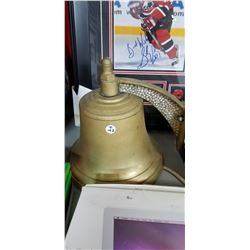142 - Shelf Two
CDC Auctions
Shelf Two
Bidding Has Concluded

Sold to f***r for (90.00 + 11.70) x 1 = 101.70143 - Shelf One
CDC Auctions
Shelf One
Bidding Has Concluded

Sold to f***r for (160.00 + 20.80) x 1 = 180.80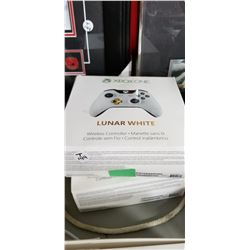144 - Shelf One
CDC Auctions
Shelf One
Bidding Has Concluded

Sold to f***r for (45.00 + 5.85) x 1 = 50.85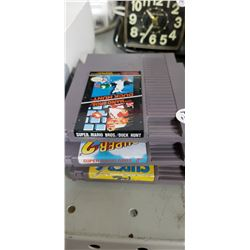145 - Shelf One
CDC Auctions
Shelf One
Bidding Has Concluded

Sold to f***r for (30.00 + 3.90) x 1 = 33.90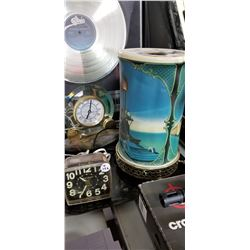146 - Shelf One
CDC Auctions
Shelf One
Bidding Has Concluded

Sold to f***r for (37.50 + 4.88) x 1 = 42.38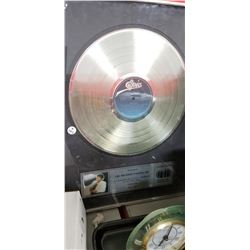147 - Shelf One
CDC Auctions
Shelf One
Bidding Has Concluded

Sold to f***r for (200.00 + 26.00) x 1 = 226.00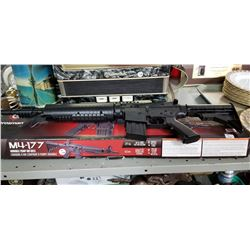148 - Shelf One
CDC Auctions
Shelf One
Bidding Has Concluded

Sold to f***r for (45.00 + 5.85) x 1 = 50.85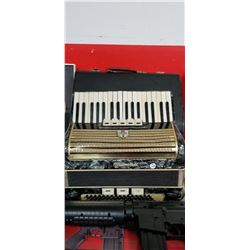149 - Shelf One
CDC Auctions
Shelf One
Bidding Has Concluded

Sold to f***r for (75.00 + 9.75) x 1 = 84.75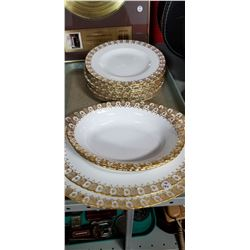150 - Shelf One
CDC Auctions
Shelf One
Bidding Has Concluded

Sold to f***r for (475.00 + 61.75) x 1 = 536.75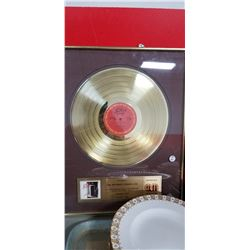151 - Shelf One
CDC Auctions
Shelf One
Bidding Has Concluded

Sold to f***r for (50.00 + 6.50) x 1 = 56.50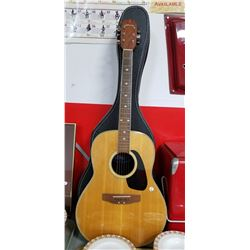152 - Shelf One
CDC Auctions
Shelf One
Bidding Has Concluded

Sold to f***r for (75.00 + 9.75) x 1 = 84.75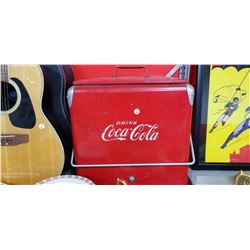153 - Shelf One
CDC Auctions
Shelf One
Bidding Has Concluded

Sold to f***r for (110.00 + 14.30) x 1 = 124.30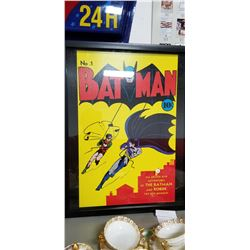154 - Shelf One
CDC Auctions
Shelf One
Bidding Has Concluded

Sold to f***r for (60.00 + 7.80) x 1 = 67.80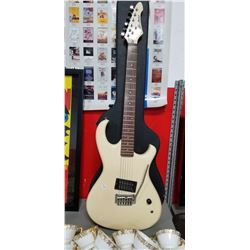155 - Shelf One
CDC Auctions
Shelf One
Bidding Has Concluded

Sold to f***r for (120.00 + 15.60) x 1 = 135.60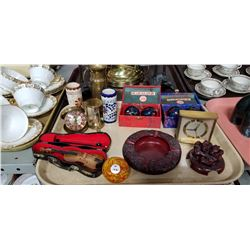156 - Shelf One
CDC Auctions
Shelf One
Bidding Has Concluded

Sold to f***r for (32.50 + 4.23) x 1 = 36.73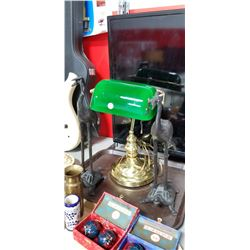157 - Shelf One
CDC Auctions
Shelf One
Bidding Has Concluded

Sold to f***r for (65.00 + 8.45) x 1 = 73.45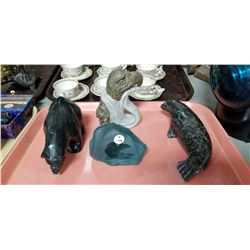158 - Shelf One
CDC Auctions
Shelf One
Bidding Has Concluded

Sold to f***r for (130.00 + 16.90) x 1 = 146.90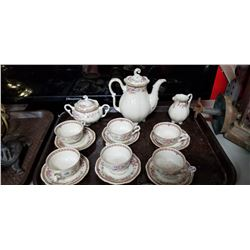159 - Shelf One
CDC Auctions
Shelf One
Bidding Has Concluded

Sold to f***r for (5.00 + 0.65) x 1 = 5.65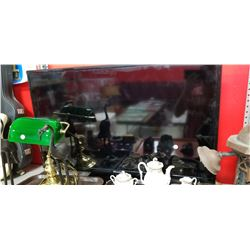160 - Shelf Two
CDC Auctions
Shelf Two
Bidding Has Concluded

Sold to f***r for (60.00 + 7.80) x 1 = 67.80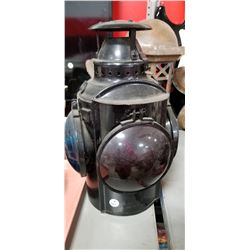161 - Shelf One
CDC Auctions
Shelf One
Bidding Has Concluded

Sold to f***r for (130.00 + 16.90) x 1 = 146.90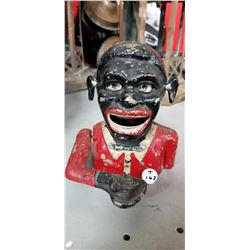162 - Shelf One
CDC Auctions
Shelf One
Bidding Has Concluded

Sold to f***r for (160.00 + 20.80) x 1 = 180.80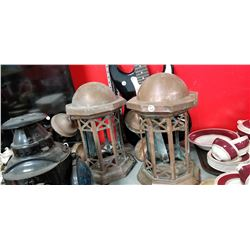163 - Shelf One
CDC Auctions
Shelf One
Bidding Has Concluded

Sold to f***r for (50.00 + 6.50) x 1 = 56.50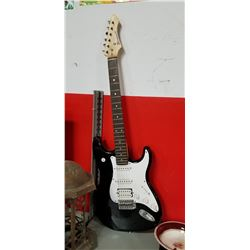164 - Shelf One
CDC Auctions
Shelf One
Bidding Has Concluded

Sold to f***r for (60.00 + 7.80) x 1 = 67.80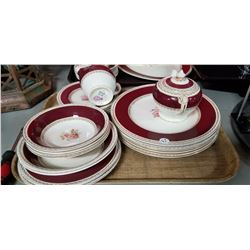165 - Shelf One
CDC Auctions
Shelf One
Bidding Has Concluded

Sold to f***r for (50.00 + 6.50) x 1 = 56.50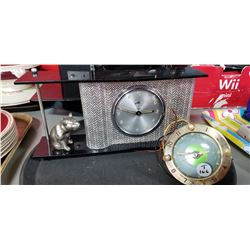166 - Shelf One
CDC Auctions
Shelf One
Bidding Has Concluded

Sold to f***r for (25.00 + 3.25) x 1 = 28.25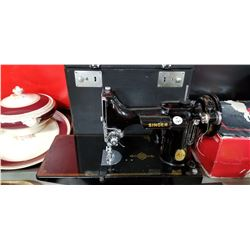167 - Shelf One
CDC Auctions
Shelf One
Bidding Has Concluded

Sold to f***r for (90.00 + 11.70) x 1 = 101.70168 - Shelf One
CDC Auctions
Shelf One
Bidding Has Concluded

Sold to f***r for (25.00 + 3.25) x 1 = 28.25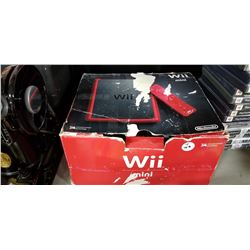169 - Shelf One
CDC Auctions
Shelf One
Bidding Has Concluded

Sold to m****3 for (40.00 + 5.20) x 1 = 45.20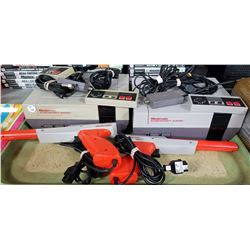170 - Shelf One
CDC Auctions
Shelf One
Bidding Has Concluded

Sold to f***r for (37.50 + 4.88) x 1 = 42.38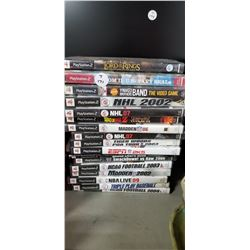171 - Shelf One
CDC Auctions
Shelf One
Bidding Has Concluded

Sold to f***r for (15.00 + 1.95) x 1 = 16.95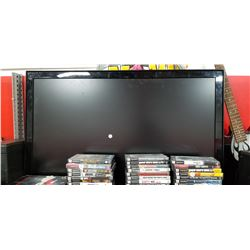174 - Shelf One
CDC Auctions
Shelf One
Bidding Has Concluded

Sold to f***r for (75.00 + 9.75) x 1 = 84.75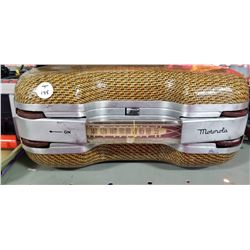175 - Shelf One
CDC Auctions
Shelf One
Bidding Has Concluded

Sold to f***r for (45.00 + 5.85) x 1 = 50.85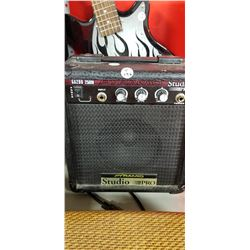176 - Shelf One
CDC Auctions
Shelf One
Bidding Has Concluded

Sold to f***r for (12.50 + 1.63) x 1 = 14.13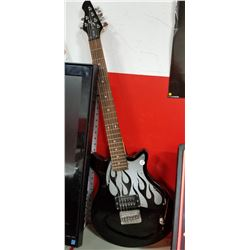177 - Shelf One
CDC Auctions
Shelf One
Bidding Has Concluded

Sold to f***r for (45.00 + 5.85) x 1 = 50.85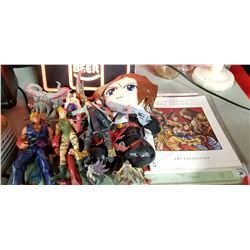178 - Shelf Two
CDC Auctions
Shelf Two
Bidding Has Concluded

Sold to f***r for (40.00 + 5.20) x 1 = 45.20179 - Shelf One
CDC Auctions
Shelf One
Bidding Has Concluded

Sold to m********s for (40.00 + 5.20) x 1 = 45.20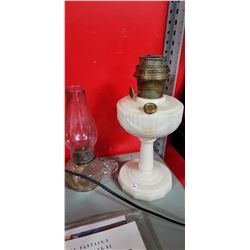180 - Shelf One
CDC Auctions
Shelf One
Bidding Has Concluded

Sold to f***r for (22.50 + 2.93) x 1 = 25.43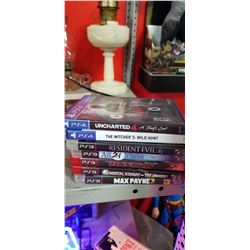181 - Shelf One
CDC Auctions
Shelf One
Bidding Has Concluded

Sold to f***r for (45.00 + 5.85) x 1 = 50.85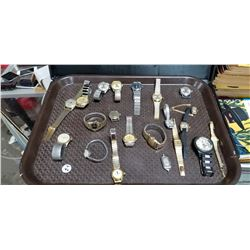182 - Shelf One
CDC Auctions
Shelf One
Bidding Has Concluded

Sold to f***r for (150.00 + 19.50) x 1 = 169.50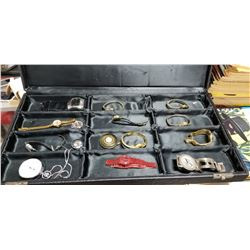183 - Shelf One
CDC Auctions
Shelf One
Bidding Has Concluded

Sold to f***r for (37.50 + 4.88) x 1 = 42.38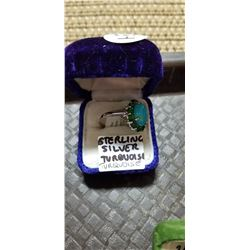185 - Shelf One
CDC Auctions
Shelf One
Bidding Has Concluded

Sold to f***r for (45.00 + 5.85) x 1 = 50.85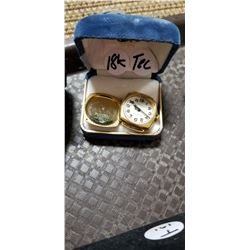187 - Shelf One
CDC Auctions
Shelf One
Bidding Has Concluded

Sold to f***r for (450.00 + 58.50) x 1 = 508.50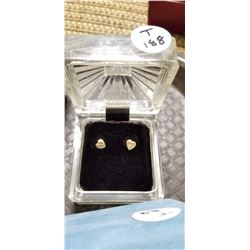188 - Shelf One
CDC Auctions
Shelf One
Bidding Has Concluded

Sold to f***r for (17.50 + 2.28) x 1 = 19.78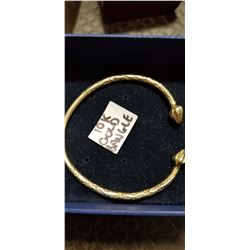189 - Shelf One
CDC Auctions
Shelf One
Bidding Has Concluded

Sold to f***r for (300.00 + 39.00) x 1 = 339.00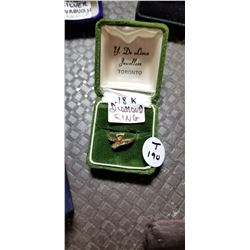190 - Shelf One
CDC Auctions
Shelf One
Bidding Has Concluded

Sold to f***r for (100.00 + 13.00) x 1 = 113.00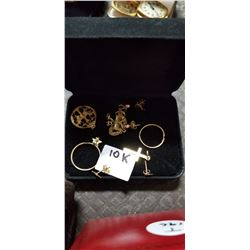191 - Shelf One
CDC Auctions
Shelf One
Bidding Has Concluded

Sold to f***r for (65.00 + 8.45) x 1 = 73.45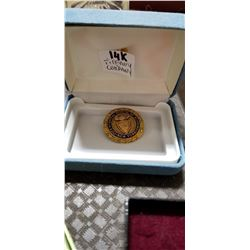192 - Shelf One
CDC Auctions
Shelf One
Bidding Has Concluded

Sold to f***r for (200.00 + 26.00) x 1 = 226.00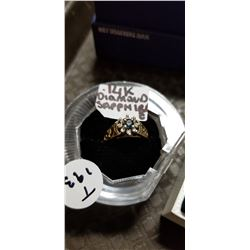193 - Shelf One
CDC Auctions
Shelf One
Bidding Has Concluded

Sold to f***r for (110.00 + 14.30) x 1 = 124.30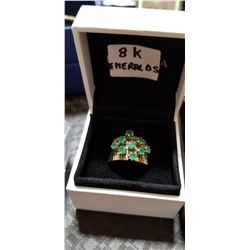194 - Shelf One
CDC Auctions
Shelf One
Bidding Has Concluded

Sold to f***r for (150.00 + 19.50) x 1 = 169.50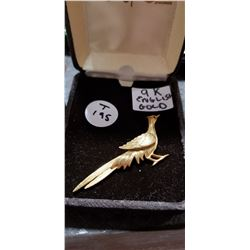195 - Shelf One
CDC Auctions
Shelf One
Bidding Has Concluded

Sold to f***r for (95.00 + 12.35) x 1 = 107.35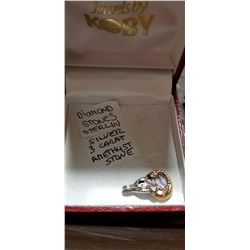196 - Shelf Two
CDC Auctions
Shelf Two
Bidding Has Concluded

Sold to f***r for (17.50 + 2.28) x 1 = 19.78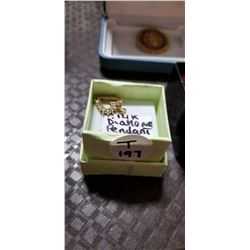197 - Shelf One
CDC Auctions
Shelf One
Bidding Has Concluded

Sold to f***r for (40.00 + 5.20) x 1 = 45.20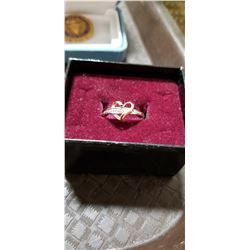198 - Shelf One
CDC Auctions
Shelf One
Bidding Has Concluded

Sold to f***r for (50.00 + 6.50) x 1 = 56.50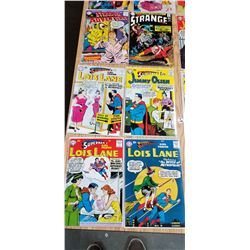199 - Shelf One
CDC Auctions
Shelf One
Bidding Has Concluded

Sold to f***r for (65.00 + 8.45) x 1 = 73.45